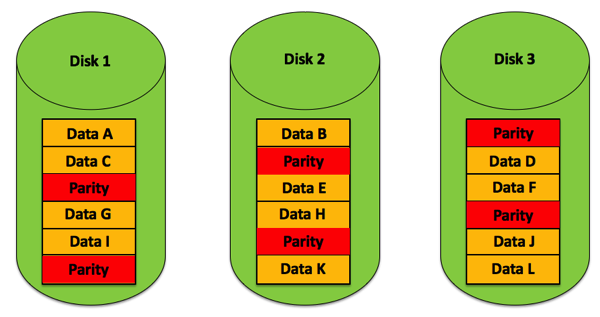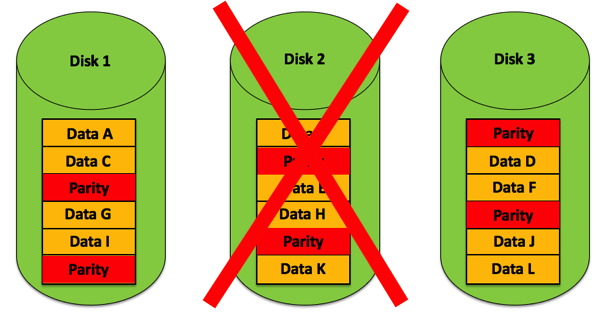# How to calculate RAID 5 Parity Information

I have previously ranted a few times about why a RAID 5 is a terrible idea for storing your Transaction Log, and why it is also very risky to store your data files on a RAID 5 volume. But in today’s blog post I want to show you how the RAID 5 parity information is actually calculated, and how it can be used to reconstruct the data in the case of a failure.

### RAID 5 – the basics

In a RAID5 array you need at least 3 disks where one disk stores the so-called Parity Information. The following picture shows this concept.The parity information is calculated during the writing, and can be used to reconstruct the data when one disk crashes. That’s pretty amazing: if one of the disks in the RAID 5 array crashes, the lost data can be reconstructed using that parity information.The question is now quite interesting: how is that parity information calculated so that lost data can be recreated with it? The trick behind it is quite simple: it uses a so-called XOR calculation. An XOR gate is one of the most primitive electronic logic gates. The following table shows you the truth table of the XOR logic gate:

 Input A Input B Result 0 0 0 0 1 1 1 0 1 1 1 0

As you can see from the truth table, the output of the XOR logic gate is only 1 if both inputs are different. If both inputs are the same, the output of the XOR logic gate is 0. And that’s already everything that you need to know to be able to calculate parity information in a RAID 5 array.

### Parity Calculation

Let’s have a look now at how the RAID 5 parity information can be calculated. Imagine we have 3 disks, and one disk stores the parity information for us. Calculating the parity information is quite simple: you just apply a XOR operation between the data that is stored on the other 2 disks.

Let’s imagine that we want to store on the first disk the character ‘a’ and on the second disk the character ‘b’. This means now that we have to store on the third disk in our RAID 5 the calculated parity information. Let’s calculate the parity information for the values ‘a’ and ‘b’ with T-SQL:

```-- Parity Calculation
SELECT ASCII('a') ^ ASCII('b')
GO
```

The result of this calculation is the value 3. Let’s do that XOR operation manually between both ASCII values of the characters ‘a’ and ‘b’:

```0110 0001 (Decimal 97 = ‘a’)
0110 0010 (Decimal 98 = ‘b’)
==============================
0000 0011 (Decimal 3 – Parity)
```

Our third disk would now store the parity information of 0000 0011 – the decimal value 3. And based on this parity information we can now reconstruct the data on the first and second disk, if one of these 2 disks crashes. Reconstructing the lost data in a RAID 5 is quite simple: we just perform a XOR operation between the still remaining data and the parity information, because:

• A XOR B = Parity
• A XOR Parity = B
• B XOR Parity = A

Let’s prove this concept again, and imagine we have lost the first disk where the value ‘a’ was stored:

```-- Reconstruct the data based on the parity information
SELECT CHAR(ASCII('b') ^ 3)
GO
```
```0110 0010 (Decimal 98 = ‘b’)
0000 0011 (Decimal 3 – Parity)
==============================
0110 0001 (Decimal 97 = ‘a’)
```

The XOR operation between the value ‘b’ and our parity information returns the value ‘a’ – the lost data! On the other hand, when we have lost the second disk where the value ‘b’ was stored, we can still reconstruct the data with the parity information:

```-- Reconstruct the data based on the parity information
SELECT CHAR(ASCII('a') ^ 3)
GO
```
```0110 0001 (Decimal 97 = ‘a’)
0000 0011 (Decimal 3 – Parity)
==============================
0110 0010 (Decimal 98 = ‘b’)
```

The XOR operation between the value ‘a’ and the parity information returns the value ‘b’. This is awesome! We have done the parity calculation just for one byte (8 bits) here, but in reality a RAID 5 controller is doing that work based on the Stripe Unit Size which is normally at least 64kb large.

### Parity Recalculation

Until now everything was easy. Imagine now you want to change existing data in a RAID 5 array. In this case you would want to recalculate the parity information with the least amount of work. From a performance perspective it would be terrible if you had to read all the data of a stripe to recalculate the new parity information when you change existing data.

Therefore you can just recalculate the parity information by accessing the old data and the parity information. All the other data on the other stripes doesn’t need to be processed. You can apply here the following XOR operation:

OldData XOR NewData XOR OldParity = NewParity

This approach is quite impressive, because all the other data stored on the other disks will not be read or processed. Imagine we want to change the character ‘a’ to ‘c’ in our example. We have to recalculate the parity information like this:

```-- Change 'a' to 'c'
-- Recalculate parity information
-- OldData XOR NewData XOR OldParity = New Parity
-- 'a' XOR 'c' XOR 3 = 1
SELECT ASCII(CHAR(ASCII('a') ^ ASCII('c') ^ 3))
GO
```
```0110 0001 (Decimal 97 = ‘a’)
0110 0011 (Decimal 98 = ‘c’)
===================================
0000 0010
0000 0011 (Decimal 3 – old Parity)
===================================
0000 0001 (Decimal 1 – new Parity)
```

The other character value ‘b’ (which was stored on our second disk) wasn’t read here – that’s very important! The result of that XOR operation is the decimal value of 1, which is our new parity information! And based on the new parity information we can again calculate the other lost data – in our present case the character values ‘c’ and ‘b’.

```-- 'c'
SELECT CHAR(ASCII('b') ^ 1)
GO

-- 'b'
SELECT CHAR(ascii('c') ^ 1)
GO
```

### Summary

The first time that you look at a RAID 5, you think that some magic is involved, because based on the parity information you can reconstruct 2 different pieces of information. But when you look at the details at the low level you can see that there is no magic – it’s a simple XOR logic operation that makes everything possible.